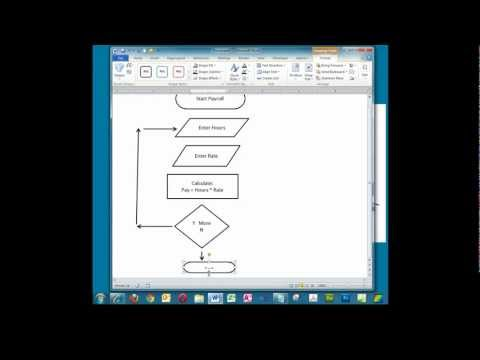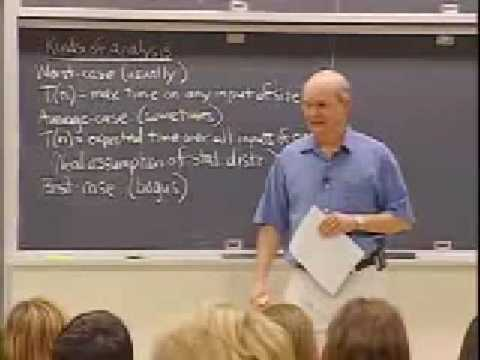## Conversion of algorithm/flowchart 2

Subject: Computer Basics

#### Overview

This note is second example of algorithm/flowchart conversion
##### Conversion of algorithm/flowchart 2
Problem: Find the largest number in an unsorted list of numbers

Let's say the input consists of a list of numbers, and this list is called L. The number L1 would be the first number in the list, L2 the second number, etc. And we know the list is not sorted - otherwise the answer would be really easy. So, the input to the algorithm is a list of numbers, and the output should be the largest number in the list.

Step 1: Let Largest = L1
Step 2: For each item in the list:
Step 3: If the item is greater than Largest:
Step 4: Then Largest = the item
Step 5: Return Largest##### Things to remember

• It includes every relationship which established among the people.
• There can be more than one community in a society. Community smaller than society.
• It is a network of social relationships which cannot see or touched.
• common interests and common objectives are not necessary for society.
##### Videos for Conversion of algorithm/flowchart 2##### Creating a Simple Flowchart in Microsoft Word.##### Lec 1 | MIT 6.046J / 18.410J Introduction to Algorithms (SMA 5503), Fall 2005

An algorithm is a sequence of steps required to solve a particular problem. The concept of algorithm was given by ALKHOWARISMI.

1. Some characteristic of an algorithm are listed below:
2. Each and every instruction should be precise and unambiguous.
3. An algorithm should have finite number of steps.
4. An algorithm should produce correct result.
5. An algorithm should not use particular programming language.
Flowchart is a graphical representation of program solving steps so that it makes clear about the program. The concept of flowchart was given by John Vonn Neuman 1945.
Types of flowchart are:
a) System Flowchart b) Program Flowchart
The common guidelines (properties) of flowchart are:
The flowchart should have only one start and ending points.
Flow lines shouldn't intersect each other.
Flowchart should not contain the programming language.
The flowchart should be neat and clear for the user.

Some of the symbols used in flowchart along with their meanings are listed below: